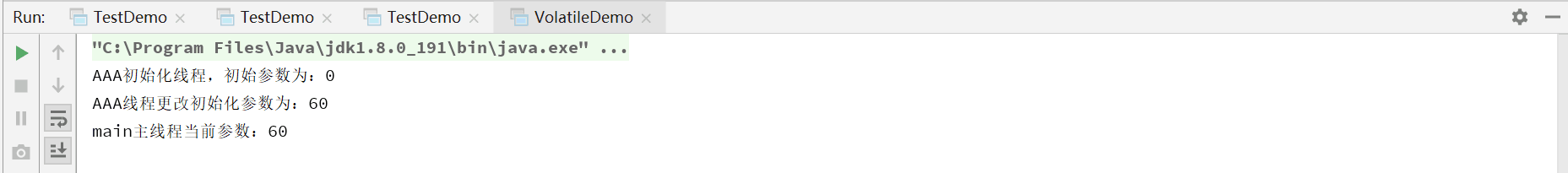使用volatile怎么验证线程之间的可见性import java.util.concurrent.TimeUnit;

class MyData{
//定义初始参数，volatile关键字使用为重点
volatile int number = 0;
//更改初始参数
public void changeNumber(){
this.number = 60;
}
}
public class VolatileDemo {
public static void main(String[] args) {
//初始化资源类
MyData myData = new MyData();
//AAA线程暂停三秒后
try{
TimeUnit.SECONDS.sleep(3);
}catch(Exception e){
e.printStackTrace();
}
//AAA线程更改初始化参数为60
myData.changeNumber();
},"AAA").start();

while(myData.number == 0){
//第二个线程是main线程，在number=0时则一直陷入死循环，当number=60时则打印主线程语句。
}
}
}

1.初始参数number未加上volatile：2.初始参数number加上volatile：volatile是JAVA虚拟机提供的轻量级的同步机制，volatile三大特征：保证可见性，不保证原子性，禁止指令重排。